Systematic approach to the correction of intonation in wind instruments

Richard A. Smith

Boosey and Hawkes Ltd, Edgware, Middlesex, UK and Department of Physics, Southampton University
Geoffrey J. Daniell
Department of Physics, Southampton University, Southampton SO9 5NH, UK

 The effect of perturbations in the bore diameter on the intonation of wind instruments is investigated. From measurements of the bore and the amplitude of the standing waves, a modified bore shape is calculated to give any desired correction in intonation.

Musical instruments have evolved over the centuries very much by trial and error. In spite of scientific investigation, many undetermined factors critically affect the performance of instruments, and empirical methods of design are still necessary. Some features, however, are easily amenable to quantitative treatment, and we present here the results of some calculations and experiments on improving the intonation and tone colour of the trumpet.
All brass instruments have acoustical and mechanical inadequacies for which most players automatically compensate1. To the average listener an instrument may sound perfectly in tune but this largely results from the competence of the player who may be able to 'lip' a note by ± 1 semitone, usually at the expense of tone colour. Similarly, the intonation of inexperienced players tends to be more affected by the deficiencies of their instruments.
The frequency of a note produced by a trumpet is determined by a complex interaction between the vibrating air column in the instrument and the player's lips. It is also affected by acoustical feedback to the player's ears, and differences of more than 10 cents (1 cent = 0.01 tempered semitone) occur when different players use the same instrument. The frequency is, however, always very close to a resonance frequency of the air column in the trumpet.
The necessity of first eliminating the contribution of the individual player to the intonation has been recognized by several workers2-7, but no satisfactory method of artificial excitation has yet been described. We have used a modification of the method originally described by Webster3 to measure the resonance frequencies of the air column in the trumpet. The apparatus (Fig. 1) operates over the frequency range C2 (65 Hz) to B7 (3,951 Hz) (C4 = middle C) and includes an automatic locking device for the location and tracking of resonance peaks. It gives a digital display and printed record of the amplitude and intonation of each resonance (in cents) relative to the equitempered scale.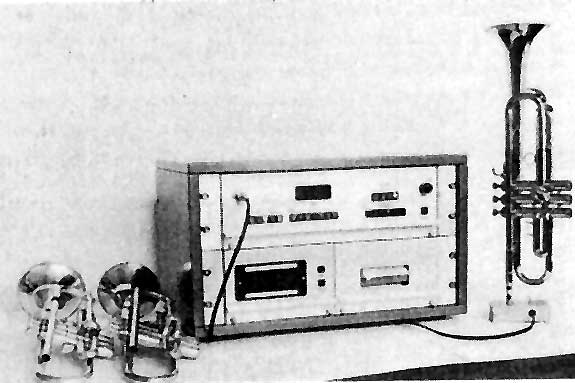Fig. 1 The apparatus designed to locate automatically and track the resonances of a wind instrument.
Digital displays indicate the intonation and amplitude of the resonances.

It was found that the frequency of each resonance of an instrument corresponded closely with the mean of the frequencies produced for this note by a number of players, so that observations with this apparatus can therefore be used for the basis of quantitative measurement of the intonation of the instrument.

Resonances and tone colour
The resonance frequencies are principally determined by the shape of the bore of the trumpet, and evolution has produced a bore shape with resonances fairly close to the appropriate notes of the equitempered scale. Unfortunately intonation is not the only consideration in fixing the resonances as the following example shows.
Figure 2 shows the first 10 resonances of the tube with their corresponding equitempered frequencies.

Fig. 2
The first 10 resonances of a trumpet with their associated equitempered frequencies. *Not normally used.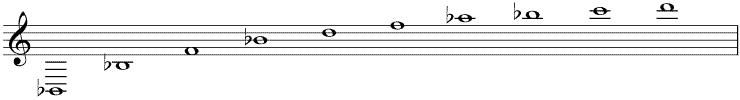Resonance 1* 2 3 4 5 6 7* 8 9 10 Note Bb2 Bb3 F4 Bb4 D5 F5 Ab5 Bb5 C6 D6 Frequency (Hz) 116.54 233.08 349.23 466.16 587.33 698.46 830.61 932.33 1046.5 1174.7

When the trumpet is sounding a steady note, D5 (587.33 Hz), the frequency of the second harmonic of this fundamental is 1,174.7 Hz, and this strongly excites the resonance at 1,174.7 Hz. This resonance is also near the fourth harmonic (at 1,165.4 Hz) of the fundamental Bb3 (233.08 Hz). But because this harmonic misses the resonance peak by 14 cents, its strength is not so strongly reinforced, and this has a significant effect on the tone colour of Bb3
In practice, the harmonics of any note fall near but not exactly on the resonances of the tube, and are, to varying degrees, reinforced by resonance. The resonances strongly affect the tone colour of notes, and hence the choice of a set of resonances is inevitably a compromise between their intonation when used as fundamentals, and their reinforcement of the harmonics of other notes. We are not concerned here with this compromise, but we show how the design of the bore shape can be modified to move selected resonance frequencies by small amounts to desired values.

Perturbations in the bore
It has been known for many years that small changes in the bore cross section near a node or antinode of the standing wave will change the resonance frequency. A decrease in area at a pressure antinode produces an increase in that frequency, and an increase in area gives a frequency decrease. At a pressure node the effects of changing the area are reversed. We have used perturbation theory to calculate the change in a given bore shape necessary to produce prescribed changes in each of the resonance frequencies.
The pressure oscillations Pn(x) at frequency fn in a standing wave in the air column in a horn, of cross section S(x), at a distance x from one end, are described by the approximate wave equation8where c is the velocity of sound. The boundary conditions at the mouthpiece and open end are assumed to be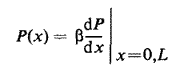where the constant b may be different at the two ends of the instrument but the values are assumed to be independent of the mode of oscillation. This is a standard Sturm-Liouville equation and the solutions form a complete orthonormal set9.
If the cross section S(x) is perturbed by a small change dS(x) and s(x) = dS(x)/S(x) the first-order change in the resonance frequencies can be shown to be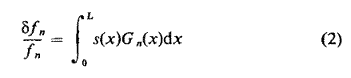where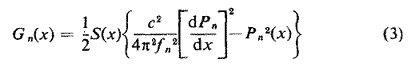In deriving this, it is necessary to take dS zero at both ends of the instrument so that the boundary conditions are not changed. The values of Pn(x) used above are taken to be normalised so that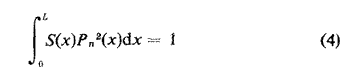This implies that the dimensions of P are L-3/2, and not those of pressure. An alternative expression for Gn(x) can be derived using equation (1)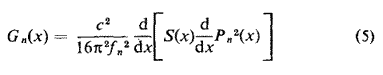We wish to prescribe dfn and calculate dS(x). It is clear that the solution is not unique and we shall seek the 'smoothest' change in the bore that produces the required frequency shifts. It may be inconvenient to make changes over the whole instrument, because of the valves and tuning slides, and we shall restrict them to the section x = 0 to x = l. To avoid discontinuities in the bore we need dS(0) = 0 and dS(l) = 0.
A suitable measure of smoothness is the mean square derivativeand we minimise this, subject to the constraints that s(x) produces the required frequency shifts using Lagrange's method of undetermined multipliers, that is we minimise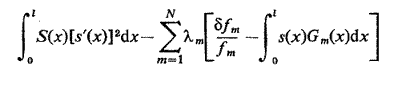The Euler equation for this problem is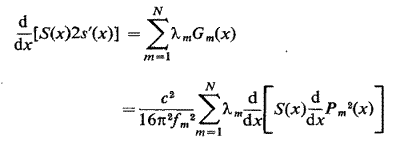Integrating twice givesA and B are arbitrary constants of integration and they can be chosen to make dS(0) = 0 and dS(l) = 0, and mm is a multiple of lm.
Substituting this into (2) gives N linear equations which, together with the conditions on dS, determine the N+2 constants mm , A, B. Equation (6) then gives the required change in bore.
The reader may wonder why we have used the 'smoothest' perturbation rather than the 'smallest' one. If we define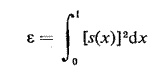and find the function s(x) that minimises this and produces the required frequency shifts we get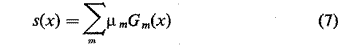This cannot, however, be used as a solution to our problem as it does not ensure that dS(0) = 0 and dS(l) = 0.

The eigenfunctions for the standing waves

To perform the calculations of the previous section the functions Pn(x) are required. Although it is possible, in principle, to calculate these given the cross-sectional area of the trumpet, it is undoubtedly easier to observe them.
In a standing wave, when damping is very small, the oscillations have the same phase at all points between adjacent nodes, and the phase changes by p across each node. The result is not exact if damping is included, but as we have neglected damping, even in equation (1), there is no advantage in observing the phase of the pressure oscillations, and we assume the phase is 0 or p. The function Pn(x) is therefore given by the observed pressure amplitude between alternate pairs of nodes and minus the observed pressure in the gaps.
The derivative dPn/dx is also required. Because of the well known dangers of numerical differentiation we obtain this by integrating equation (1) giving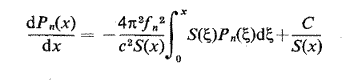The value of the constant of integration C is found by integrating again, thus introducing another constant, and finding both constants by satisfying the equations at two particular values of x.

Varying the length of pipe over which the perturbation is made
An interesting result can be derived about the mean square perturbation, which can be evaluated using equation (7). This can be differentiated with respect to l, bearing in mind that the numbers m depend on l. The derivatives of m can be eliminated by differentiating equation (2) and we get the remarkable result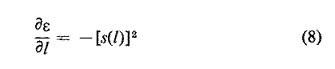It follows that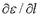is never positive, and the mean square value of the perturbation required always decreases as we spread the perturbation over longer lengths of the tube.

Asymptotic results
The high eigenvalues of a Sturm-Liouville equation have simple asymptotic distributions9. When n is large, fn ~ nc/2L andin which qn depends on the boundary conditions. A musical instrument like the trumpet has evolved into a shape such that the frequencies of the resonances are almost uniformly spaced. In other words, the formula fn ~ nc/2L, which can be proved for large n, is in fact almost exact for all n. This suggests that the associated asymptotic eigenfunctions, Pn(x), might be good approximations for all n. This would avoid the difficult measurement of Pn(x). If the boundary conditions in the trumpet are approximately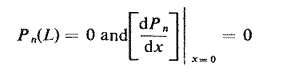we can show that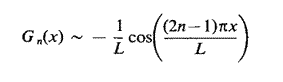The integrals involved in the calculations can now be evaluated analytically.
We have tested the usefulness of this by prescribing some values for dfn, calculating the bore shape required using the asymptotic expression for Gn(x) and then calculating the exact frequency shifts (equation 2) that this bore shape would produce, using the correct observed value of Gn(x). The changes produced are found to be in the right direction, but the magnitude of error is large. The asymptotic results can, however, be usefully employed if (a) a rapid approximate answer is required: the measurement of Pn(x) is time consuming, or (b) if a very high harmonic needs adjustment, where the observations of the resonance are impossible.

Verification of the theory
The trumpet was driven by the automatic tuner described in the first section and the sound pressure was measured in two ways; using probe tubes inserted radially through holes in the bore at each centimetre of the instrument's length and using a long flexible probe drawn through the instrument. There was little variation between the results of either method and they are shown with the computed functions Gn(x) in Fig. 3.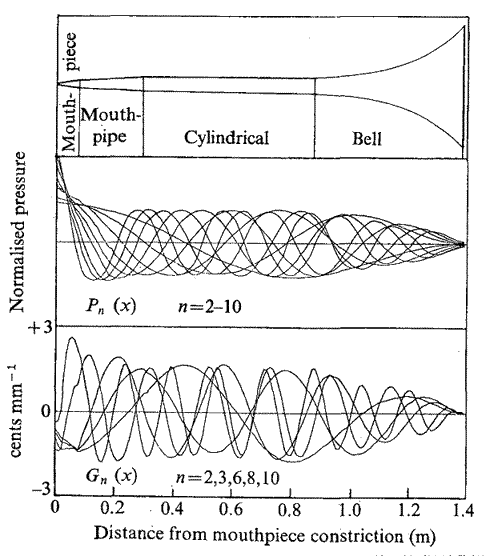Fig. 3 The physical shape of a trumpet (not to scale) with Pn(x) and Gn(x) for selected modes.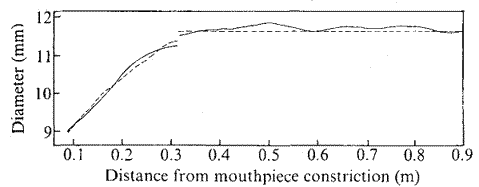Fig. 4 The bore shape of a trumpet (conical and cylindrical portions)
before and after perturbation:. ____, perturbed bore; ----, original bore.

An earlier section has indicated the difficulties involved in deciding upon the optimum intonation of an instrument. To verify our ideas, however, these arbitrary changes were chosen: for the fifth and tenth order resonance, a change of +5 cents, for the sixth order resonance a change of -5 cents, and all other resonances to be unaltered. Similarly, the perturbed length was arbitrarily restricted to the first 0.9 m of the instrument.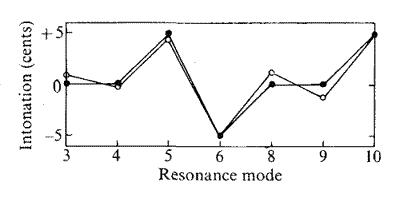Fig. 5 Relative intonation of seven resonance modes.
• , Required change in intonation; o, change obtained by perturbation.

This bore provides the 'open' notes of the instrument and additional tubing is inserted by valves to produce a chromatic scale. Consequently the effect of our perturbation is likely to be less accurate as more tubing is added; the perturbation method could be applied to this valve tubing for further correction to notes using valve tubes. Equation (8) implies that because of the relatively short length of the valve tubing, only small intonation corrections could be made in this way without unacceptably large diameter changes.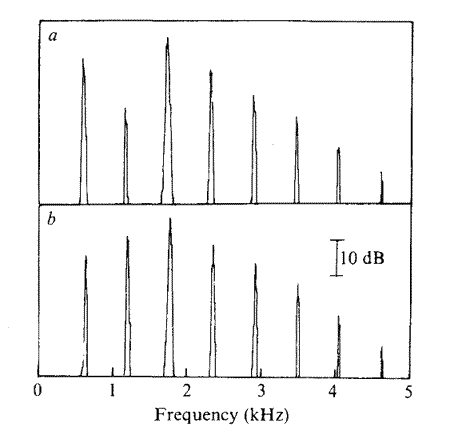Fig. 6
The spectra of note D5 (587.33 Hz) blown by a professional musician,
(a) before, and (b) after perturbation.
Both notes were played mezzo forte in an anechoic chamber
with the microphone at 1 m along the axis of the instrument.

A graph of the new bore shape (Fig. 4) indicates a maximum change in diameter of < 0.2 mm. This may be compared with a maximum of 0.14 mm for the smallest mean square perturbation mentioned earlier.
The new shape was reproduced with glass reinforced plastic and its resonances measured using the automatic tuner. There is close correspondence between the required and measured intonation (Fig. 5); the small errors mainly arise from the difficulty in producing a new bore to an accuracy of better than 0.02 mm.
It can be appreciated from the qualitative effects of diameter changes at nodes and antinodes that some prescribed frequency shifts may require conflicting diameter changes. The result in these cases is that very large increases and decreases in diameter are required over short distances. These would be unacceptable for a musical instrument and would also render our first-order perturbation theory invalid. Our optimisation procedure makes sure that large changes in diameter are not used unnecessarily.
For comparison another calculation has been made which assumes a non-optimum bore shape given by a superposition of sine waves. The maximum diameter change required here was 3 mm.

Curing a faulty trumpet
Although the foregoing theory is not ideally suited to the complete acoustical design of a new instrument, the best application of this work is with the improvement of the individual notes of prototype or production instruments. One such instrument was found to have a weak second harmonic when playing D5 (587.33 Hz) (Fig. 6a). As already mentioned, this harmonic uses the tenth resonance for reinforcement; and this was found to be flat with respect to the fifth. The tenth resonance is not normally played as a fundamental and hence can be altered to improve the tone colour. Using our new technique we were able to raise this tenth resonance by ten cents to give the improved response (Fig. 6b).
This work was supported in part by the SRC and Boosey & Hawkes Ltd. We thank Dr D. M. A. Mercer for encouragement and also acknowledge the assistance of Mr W. Tompkins and the trumpet player, Michael Laird.

Received May 13; accepted July 21, 1976 and published in Nature, Vol. 262, August 26, 1976, pp. 761-765.

1 Smith, R. A., thesis, Univ. Southampton (1974) .
2 Martin, D. W., thesis, Univ. Illinois (1941).
3 Webster, J. C, J. acoust. Soc. Am., 19, 902-906 (1947).
4 Igarashi, J., and Koyasu, M., J. acoust. Soc. Am., 25, 122-128 (1953).
5 Hunt, F. V., J. acoust. Soc. Am., 10, 216-227 (1939).
6 Backus, J., and Hundley, T. C, J. acoust. Soc. Am., 49, 509-519 (1970).
7 Benade, A. H., and Jansson, E. V., Technical Report (Speech Transmission Laboratory, Royal Institute of Technology, Stockholm, 1973).
8 Morse, P. M., Vibration and Sound, 2nd ed., 269 (McGraw-Hill, New York, 1948).
9 Morse, P. M., and Feshbach, H., Methods of Theoretical Physics, par. 6.3 (McGraw-Hill, New York, 1953)